D. DISPERSION MODELING SYSTEMS

1. VLSTRACK

a. Model Basics. VLSTRACK (Bauer, 1998) is a Lagrangian transport and dispersion model employing the Gaussian puff method, in which a collection of three-dimensional puffs represents an arbitrary concentration field. To solve the dispersion model equations, a continuous source is simulated by releasing pollutant puffs at regular intervals over the duration of the release.

b. Meteorological Data Fields. Input files can be in these different forms: (1) time variable assumes uniform meteorological conditions over relatively flat terrain; (2) height-time variable meteorology also assumes uniform flow over relatively flat terrain, but allows wind vectors and air temperatures to change as a function of height; (3) latitude and longitude-time variable meteorology also applies to relatively flat terrain but allows meteorological conditions to vary spatially and temporally, such as measured by a field of meteorological towers; and (4) xyz-time variable allows full, three-dimensional variations of meteorological conditions. For the Khamisiyah analysis, VLSTRACK used the last data type.

VLSTRACK calculated the Khamisiyah dispersion based on some variables generated by the meteorological models, including the three velocity components for transport and the friction velocity and surface roughness for stability and surface layer velocity profiles. COAMPS-calculated heat flux and potential temperature were used in an external routine to compute the Monin-Obukhov length.

c. Terrain Data. VLSTRACK does not explicitly account for terrain effects because it does not generate a mass-consistent wind field internally. However, since terrain information is required when specifying meteorological data at several locations (either randomly-spaced stations or regularly-spaced grid points), the terrain is the same as the full resolution of the mesoscale weather model output. Meteorological conditions at the puff centroid are obtained by interpolating the xyz-time variable data set.

d. Mean Field Interpolation and Advection. Meteorological conditions at the puff tracking point are interpolated using the three meteorological grid locations closest to the interpolation point, followed by vertical interpolation. The model obtains the downwind and crosswind puff centroid transport by simple forward differencing: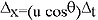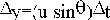(Equation A-4)

where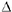x = change in downwind distance,y = change in crosswind distance,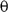= wind direction relative to grid orientation,t = time step, and u = wind speed.A fixed 30-second time step was used in the VLSTRACK runs when advecting puff elements. VLSTRACK interpolates the meteorological fields in time if the advection time step is less than the update frequency of the meteorological data.

If the wind speed at the representative puff transport height is less than 0.5 m/s, VLSTRACK uses a wind meander approach, with the wind speed set to 0.49 m/s and the wind bearing set to the random wind direction, i.e.,

ux = 0.49 cosuy = 0.49 sin(Equation A-5)

e. Dispersion Calculations. VLSTRACK uses the Monin-Obukhov similarity theory to determine the PBL’s governing parameters and describes stability in terms of the Pasquill-Gifford (PG) stability classes. In addition to the temporally and spatially variable wind fields, VLSTRACK uses meteorological model-produced friction velocity and roughness lengths to update stability parameters and perform dispersion calculations. In addition, VLSTRACK uses the heat flux calculated by COAMPS to determine the Monin-Obukhov length and the Golder (1972) nomogram to determine the PG class based on the Monin-Obukhov length and the roughness length.

If the meteorological model does not provide friction velocity, u*, it can be determined from the wind speed, ur, at the lowest measurement (or model prediction) height, zr, the Monin-Obukhov length, L, and the roughness length, z0, based on this similarity theory.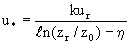(Equation A-6)

 where: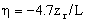stable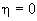neutral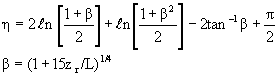unstable

k is the Von Karman constant

VLSTRACK assigns the height of the mixing layer, zr, from Table A-6 (Saucier, 1987):

Table A-6. VLSTRACK’s Specified mixing layer heights

 PG Stability Class Mixing Layer Height (m) A 1800 B 1400 C 1000 D 800 E and F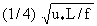(Zilitinkevich 1975), where f is the Coriolis parameter (10-4 s-1)

Parameterizations for the Lagrangian time scales and the standard deviations of the wind velocity fluctuations for relative puff dispersion are obtained from Saucier (1987) and Hanna et al. (1982). The model determines the Lagrangian time scales based on the length scale and velocity scale of the energy-containing eddies. VLSTRACK approximates these by constant values, which are only a function of the PG stability class and are shown in Table A-7.

Table A-7. Lagrangian time scales used in VLSTRACK

 PG Stability Class Lagrangian Time Scale (seconds) Lateral Vertical A 400 400 B 400 400 C 400 400 D 400 200 E 400 100 F 400 50

The model assumes the standard deviations of turbulent velocity fluctuations, denoted by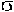u,v, andw, are functions of the friction velocity, u*.

These formulas were adapted from Hanna et al. (1982):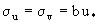(Equation A-7)

 where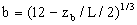unstable b = 2.1 neutral, stable and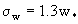where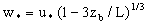unstable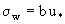where b = 1.3 neutral, stable (Equation A-8)

VLSTRACK tracks all vapor, solid, and liquid masses as separate puffs with Gaussian concentration distribution. If the characterized source type results in a line source, then the model converts the line source to a series of overlapping puffs. Liquid and solid masses are further divided into droplet and particle sizes based on the entered droplet mass-median diameter and geometric standard deviation.

Puff diffusion parameters (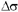2) are based on Taylor’s statistical theory. VLSTRACK currently uses these expressions for vapor puffs2 (Bauer, 1998):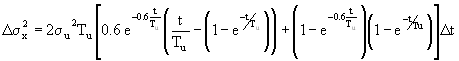(Equation A-9)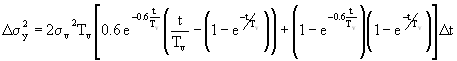(Equation A-10)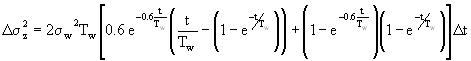(Equation A-11)

whereu,v, andw are turbulent fluctuations of the u, v, and w wind components; Tu, Tv, and Tw are the Lagrangian time scales; t is the current time; andt is the integration time step. The model includes additional factors in these equations for large droplets.

f. Vapor and Liquid Fractions. VLSTRACK normally determines the initial fractions of vapor and liquid based on an energy balance for exploding munitions. For the Khamisiyah analysis, the Dugway tests defined the initial vapor and liquid fractions of the release. Because the Dugway tests also estimated the time distribution of evaporation of agent that initially spilled on wood and soil, all dispersion models treat the release as a pure vapor release.

g. Vapor Decay and Deposition. As described before, GB and GF vapor reacts with pollutants in the air during dispersal. The rate of reaction generally follows an exponential decay form. An exponential decay rate adjustment has been added to the VLSTRACK 3.0.1 (used in the 2000 modeling) vapor transport subroutine.

The version of VLSTRACK used in the 1997 modeling handled deposition by gravitational settling only. For liquid droplet clouds, VLSTRACK allows puffs consisting of droplets or particles of 10 microns or greater in diameter to impact the ground once the puff centroid comes very close to the surface. However, the model does not allow airborne GB and GF vapor to deposit. Recent studies have suggested that the ground surface will absorb the G-agent vapor at a deposition velocity in the range of 0.1 to 2 cm/s. VLSTRACK 3.0.1 used in the 2000 modeling contains an incremental vapor deposition subroutine, where deposition is accounted for through a reflection coefficient. VLSTRACK 3.0.1 assumes a reflection fraction of 0.985, which corresponds to a deposition velocity of 0.1 cm/s.

h. Tracking and Splitting Vapor Puffs. VLSTRACK originally sets the representative transport height to the average of the puff center height and the height of interest. If this average height is more than one vertical cloud sigma from the centroid height, the representative transport height is reset to one vertical cloud sigma toward the dosage output height. During the 1997 Gulf War modeling effort, VLSTRACK based puff transport on this original methodology for ground-based puff. Dispersion model results strongly depend on the near-surface algorithm due to strong wind shear near the surface. It was found that the original VLSTRACK algorithm often produces questionable results for near-surface releases, thus the following alternative formula was used for the near-surface puff transport height, zT, in the 2000 modeling: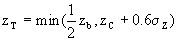(Equation A-12)

wherez is the vertical puff sigma, zb is the mixed layer height, and zc is the puff center height.

VLSTRACK may split puffs if they: (1) reach a certain size, (2) have enough mass to remain above toxic levels when split, and (3) will not create puffs exceeding the array size imposed by the model. The original puffs are removed from the computations once they are split. For the Khamisiyah analysis, the vapor puff arrays were assigned appropriate dimensions to allow enough splitting to occur to correctly represent the downwind hazard at low toxicity levels.

i. Pollutant Concentration Calculation. A Gaussian distribution approximates the concentration associated with each airborne puff. The basic equation without reflection terms is: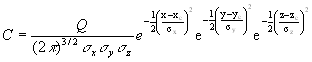(Equation A-13)

where Q is the puff mass;x,y, andz are dispersion coefficients in the x-, y-, and z-directions; and xc, yc, and zc are the puff center location.

Predicted trajectories and increasing puff size may cause puffs to intersect the ground and the top of the mixing layer . In reality, the ground surface will remove, via incremental deposition, some agent and some agent will penetrate the mixed layer. The 1997 VLSTRACK methodology did not address such incremental deposition and assumed perfect reflections from the ground surface and the inversion height. (For the 2000 modeling NSWC developed vapor decay and deposition subroutines for VLSTRACK. Appendix A, Section IV.C describes these model enhancements.) Similar to the method of images, additional terms are added to the above expression for concentration to satisfy a perfect-reflection condition at the ground and the top of the mixed layer. For a very shallow mixed layer, the puff is assumed to be uniformly distributed vertically within the layer, where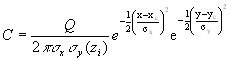(Equation A-14)

j. Concentration Analysis Grid.
VLSTRACK uses a Cartesian coordinate system to quantify its concentration fields. The origin of the output grid is the Khamisiyah Pit location, with the x-direction heading east and the y-direction heading north. VLSTRACK uses 101 x 101 horizontal grid points for the Khamisiyah analysis. The horizontal grid increments were fixed atx = 850.36 m andy = 479.99 m. In comparison, the highest-resolution meteorological grid spacing in COAMPS was 5 km. VLSTRACK has the option of using an evolving grid, where the grid is expanded with the evolving hazard.

2. HPAC/SCIPUFF

a. Model Basics. HPAC/SCIPUFF (Sykes et al., 1998; DSWA, now DTRA, 1997) is a Lagrangian dispersion model. As with VLSTRACK, HPAC/SCIPUFF is based on a Gaussian representation. However, in contrast to other models, HPAC/SCIPUFF applies a generalized moment representation where moments up to second-order (i.e., Q,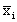, andij) are used to describe the Gaussian distribution: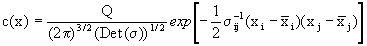(Equation A-15)

Using an angle bracket to denote an integral over all space, the spatial moments are defined as: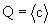zeroth moment (mass) (Equation A-16)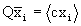first moment (centroid) and (Equation A-17)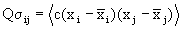second moment (spread) (Equation A-18)

HPAC/SCIPUFF solves transport equations for individual puff moments based on a linear representation for the local velocity field: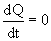(Equation A-19)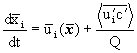(Equation A-20)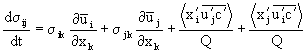(Equation A-21)

where these equations are derived by forming successive moments of the scalar transport equation (before Reynolds averaging). The second term on the right side of Equation A-20 is the turbulent flux. The first two terms on the right of Equation A-21 represent shear distortion and the last two terms represent turbulent diffusion. HPAC/SCIPUFF predicts the concentration mean and variance, required for probabilistic output, through a second-order turbulence closure solution of the advection-diffusion equation (see Sykes et al., 1998, for more details).

b. Meteorological Input. For the Khamisiyah modeling, HPAC/SCIPUFF required both three-dimensional meteorological mean fields (e.g., wind and temperature) and two-dimensional boundary layer parameters (e.g., the friction velocity, surface roughness, and sensible heat flux.) These fields were provided by MM5, COAMPS, and OMEGA. To increase computational efficiency, the complete meteorological fields provided by mesoscale models were reduced to a set of 400 profiles centered at Khamisiyah. The original vertical resolution of the meteorological models was preserved in these profiles. The spacing between profiles is comparable to the interval of COAMPS Grid 2 (15 km). The resulting dispersion modeling domain is approximately 300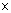300 km.

c. Terrain Data and Mass Consistency. The terrain data was used directly from the HPAC distribution CD-ROM. HPAC 3.1 assumed all surface characteristics are spatially uniform (Version 3.2 included a 1 km land-use data base). HPAC invokes either the HPAC Mass Consistent wind model (MC-SCIPUFF) or the more advanced Stationary Wind Fit and Turbulence (SWIFT) model to prepare a gridded wind field consistent with terrain. Even though SWIFT is more advanced, it allowed only a maximum of 25 profiles, whereas MC-SCIPUFF allowed a maximum of 400 profiles. Therefore, MC-SCIPUFF was used for all Khamisiyah analyses.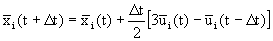(Equation A-22)

Mean meteorological fields are updated every large time step,tL, using simple linear interpolation. If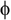(t) represents a field at time t, then the time interpolation is defined by: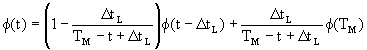(Equation A-23)

where TM is the next time step when the fields are given. HPAC/SCIPUFF enhances efficiency in calculating puff transport by implementing adaptive time stepping. Each puff uses a time step appropriate for resolving its local evolution rate, so the model does not have to use a small step for the entire calculation. HPAC/SCIPUFF uses bilinear interpolation to compute the mean wind and temperature at a puff centroid.

e. Planetary Boundary Layer Parameters. To a large extent, parameters including the surface roughness length, z0; the boundary layer depth, zi; the Monin-Obukhov length, L; and the friction velocity, u* , define the mean wind field and turbulent profiles in the planetary boundary layer (PBL). HPAC/SCIPUFF can optionally use these parameters as provided by high-resolution meteorological models to calculate atmospheric stability and dispersion. This enhances the coupling between the meteorological and dispersion fields and maintains dynamic consistency.

HPAC/SCIPUFF specifies PBL parameters according to the calculation mode chosen, including the Simple, Observation and Calculated modes. Even though mesoscale meteorological model input drives HPAC/SCIPUFF since the predictions were treated as pseudo observational profiles, HPAC/SCIPUFF was run in the Observation mode.

In this mode, HPAC/SCIPUFF directly accepts PBL turbulence parameters from external files. The 2000 Khamisiyah analysis used PBL parameters given by the high-resolution mesoscale models (OMEGA, COAMPS, and MM5). PBL parameters were derived for OMEGA and COAMPS and input to HPAC for the 1997 Khamisiyah analysis. The data to derive PBL parameters were not available for MM5, so the heat flux and mixing layer heights from OMEGA were used instead.

As implemented in HPAC 3.1 (1997 modeling), HPAC/SCIPUFF accepted only one roughness length for the entire domain. HPAC 3.2 (2000 modeling) (DTRA, 1999) includes a land-use database providing a spatially variable roughness length with a 1-km resolution.

If the surface heat flux is not available when the Observation PBL mode is specified, it can be estimated from the Pasquill-Gifford (PG) stability class, as described below. An average Monin-Obukhov length is first assumed for each stability class (Sykes and Lewellen, 1992). Table A-8 lists the relationship between the PG stability class, the Monin-Obukhov length (L), and the boundary layer depth (zi), if not already specified in inputs.

Table A-8. Relationship between PG stability class,
Monin-Obukhov length, and an assumed boundary layer depth

 PG Stability Class Monin-Obukhov Length (m) Boundary Layer Depth (m) A -5 1000 B -12.5 1000 C -50 1000 D -1000 1000 E 25 125 F 13 80

The heat flux is then calculated from: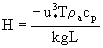. (Equation A-24)

For the Simple mode, the surface heat flux is calculated from a simple diurnal-varying formula: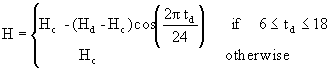(Equation A-25)

For the Calculated mode, the surface heat flux is computed using a surface energy balance method. For day-time unstable conditions, the calculation balances the net incoming radiation with the total surface heat flux and requires partitioning the surface heat flux into sensible and latent components (Holtslag and van Ulden, 1983). For night-time stable conditions, the surface fluxes are calculated based on Venkatram’s proposed scheme (1980).

f. Decay and Deposition. Activity decay can apply to any material to represent a simple reduction in viable fraction or other linear decay effects. Each puff carries an activity coefficient, fA which is initialized to unity at release time. During the transport calculation, the coefficient evolves according to the relation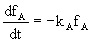(Equation A-26)

where kA is the user-specified decay rate. At each time step , the effective mass of each puff is reduced by the factor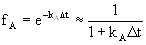(Equation A-27)

The decay rate specification includes a diurnal variation so the effects dependent on solar radiation can be described.

HPAC/SCIPUFF models gaseous dry deposition as a continuous process. The user specifies the deposition velocity, Vd, as a constant. At each time step for each puff, a sink term, FS, is calculated for inclusion in Equation A-19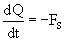(Equation A-28)

where the deposition flux at the surface is calculated by integrating the product of Vd and the mean concentration over the surface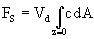(Equation A-29)

The flux Fs drives the zeroth moment equation (A-19), which is coupled with the first and second moment equations (A-20 and A-21, respectively). This modification of the moment equations is the only change to the calculations resulting from a dry deposition effect. The model retains a total reflection boundary condition at the surface, since the flux is assumed to be small.

f. Turbulence Specification. Turbulence profiles, best understood for neutral and free-convection conditions, are analytically described in terms of the primary mechanisms driving the PBL growth: the surface heat and momentum fluxes. In a neutral flow, surface heat flux effects are negligible and mechanical shear (which scales with u*) is dominant. Free convection occurs under light wind conditions with a positive surface heat flux. In this case, turbulence scales with w*, the convective velocity scale.

In HPAC/SCIPUFF the turbulence velocity correlation for the small scale turbulence of the PBL is represented using standard analytic shape functions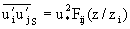(Equation A-30)

for the shear-driven component and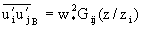(Equation A-31)

for the buoyancy-driven component. Neutral profiles are based on the work of Townsend (1976), Spalart (1989), and Mason and Thompson (1987). Free convection profiles come from Deardorff’s (1970) laboratory experiments and more recent large eddy simulation numerical experiments by Mason (1989) and Schmidt and Schumann (1989).

g. Puff Dispersion. HPAC/SCIPUFF applies a second-order closure of Reynolds-averaged equations to turbulence modeling and computes the mean and variance concentrations. This method also provides a dynamic relationship between turbulence diffusivities and velocity fluctuation statistics—the fundamental quantities of turbulence (e.g., turbulent flux correlations).

h. Probabilistic Calculations and Output. HPAC/SCIPUFF produces probabilistic output by solving the equations for the mean concentration and concentration fluctuation variance. Once the two moments (mean and variance) of the concentration fields are known, HPAC/SCIPUFF assumes the concentration probability distribution to be clipped normal, pc, i.e., replacing all negative concentration values in a general Gaussian distribution with zero values. The mean of a clipped normal distribution is related to the mean, µG, and variance,G, of a general Gaussian distribution as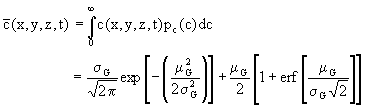(Equation A-32)

The variance of a clipped normal distribution is related to m
G and s G as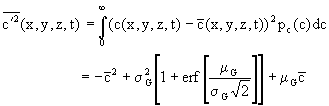(Equation A-33)

where erf is the error function. Unless otherwise noted, the HPAC/SCIPUFF results for Khamisiyah show contours for which, based on the uncertainty due to calculated turbulence levels, the probability a person standing on the contour would be exposed to a level exceeding the contour value is 1%. This tends to enlarge the area of the contour (depending on the turbulence intensity) relative to the mean contour.

i. Averaging Time. The concentration fluctuation variance predicted by HPAC/SCIPUFF depends on the velocity fluctuation statistics used to determine the dispersion rates. A meaningful quasi-deterministic prediction can sometimes be obtained by restricting the definition of the turbulent velocity fluctuations. By ignoring the large-scale meandering motions in the turbulence, a conditional average result can be generated where the uncertainty in the plume location is ignored.

Conditional average is obtained by specifying an averaging time parameter, Tav. This time scale is converted into a length scale, L av, using the local wind speed. The averaging time should be thought of as a sampling time, but also can be considered to be the release duration if this is shorter than the sampling time. The relative dispersion result corresponds to Tav = 0. Commonly used dispersion coefficients for the PG stability class for short-range dispersion from surface releases are based on an averaging time of about 10 minutes. The ensemble average result is obtained for large Tav. The model applies a reduction factor to the velocity variances if the length scale of the velocity fluctuations is larger than the conditional average scale, which is defined as the larger of L av and the instantaneous cloud scale.

Turbulent motions on scales smaller than the instantaneous cloud are always diffusive and should always be included in the conditional average. If the instantaneous cloud is smaller than the conditional average cloud, the averaging time determines the range of scales.

j. Vapor Puff Splitting and Merging. In HPAC/SCIPUFF, the size of a puff increases through turbulent diffusion and, in the case of inhomogeneous meteorology, through elongation in the wind shear direction. As the puff grows, the local representation of the turbulence and velocity fields at the puff centroid location becomes increasingly inaccurate. HPAC/SCIPUFF uses a grid-based method Sykes and Henn (1992) suggested to split puff into smaller components to more accurately sample the variation in meteorology. HPAC/SCIPUFF also merges puffs if their number becomes excessive, where merging rules also require moment conservation (Sykes et al., 1998).

k. Concentration Analysis Grid. HPAC/SCIPUFF adapts its puff tracking calculations to changes in spatial scale. Near the source, small spatial scales require small time scales to accurately resolve the dispersion process. As the plume moves downwind, the time scales increase. Figures A-20 and A-21 show the adaptive HPAC/SCIPUFF concentration grid, which provides enhanced resolution where required, at 2 hours and at 3.13 days after the release. The surface grid consists of cells which have sensed non-zero surface concentrations. Once a cell is identified with a non-zero concentration, HPAC/SCIPUFF includes it in dosage calculations for that and all subsequent times.

l. Model Output. HPAC/SCIPUFF describes the concentration field by summing contributions from each puff using the generalized Gaussian formula, (Equation A-15). Dosage calculations numerically integrate concentration with time and involve a summary updated at each time step.

2. Comparing Dispersion Models

The DoD modeling team used HPAC (version 3.1; DSWA, 1997) and VLSTRACK (version 3.0; Bauer and Gibbs, 1998) dispersion models to assess the possible hazard to US forces from an inadvertent release of nerve agent during a large-scale demolition at the Khamisiyah Pit on March 10, 1991.

Both VLSTRACK and HPAC/SCIPUFF use the COAMPS wind field; the MM5 and OMEGA fields drive HPAC/SCIPUFF only. Even though the same meteorological fields are used, the ways the dispersion models use them are different. HPAC/SCIPUFF uses a set of artificial profiles by selecting a reduced set (i.e., 400) of horizontal grid locations from the meteorological model grid. HPAC/SCIPUFF then uses the MC-SCIPUFF meteorological model to generate a mass-consistent gridded wind field based on refined surface topography. HPAC/SCIPUFF can use the data directly, and thus bypass the mass-consistency calculations, if these data are on a latitude/longitude or UTM grid. However, none of the mesoscale meteorological models used either of the grid systems. The alternative was to interpolate the profiles using the mass-consistency and achieving higher terrain resolution at the same time. VLSTRACK does not have an integrated meteorological model; its three-point interpolation scheme directly uses mesoscale meteorological fields.

Based on the similarity theory, the PBL’s mean wind and temperature profiles and turbulence are primarily functions of the surface roughness (z0), boundary layer depth (zi), Monin-Obukhov length (L), and friction velocity (u*). Both HPAC/SCIPUFF and VLSTRACK use standard tables and equations to specify u* and z0 if they are unavailable from the meteorological model outputs. For the Khamisiyah analysis, VLSTRACK uses values consistent with COAMPS. HPAC/SCIPUFF assumed that surface characteristics are spatially uniform for the 1997 analysis. HPAC/SCIPUFF used the land cover in the HPAC LANDSCAN 30 arc-second database to derive the surface roughness, Bowen ratio, and albedo for the 2000 analysis.

VLSTRACK and HPAC/SCIPUFF calculate L and zi quite differently. For example, VLSTRACK does not directly calculate or use surface heat flux values (H) in modeling the PBL, but uses the Golder nomogram to establish the primary link between the meteorological conditions (captured by the PG stability class) and the Monin-Obukhov stability characterization.

As described above, HPAC/SCIPUFF specifies the PBL parameters according to the calculation mode (Simple, Observation, or Calculated). For the Observation mode, the model either directly accepts the PBL parameters in the input file or calculates them based on the PG stability class. The latter approach is comparable to the VLSTRACK implementation. The Simple mode consists of very simple diurnally-variable formulae. The Calculated mode consists of detailed energy budget methods for determining the surface heat flux and prognostic equations for determining zi. The Calculated option was always used for the Khamisiyah analyses. However, the heat flux and the mixed-layer height were provided by the meteorological models for the 1997 and 2000 analyses, thus over-riding the internal calculations of these two PBL parameters.

VLSTRACK and HPAC/SCIPUFF apply fundamentally different puff dispersion methods. VLSTRACK implements dispersion algorithms adapted from the NUSSE4 Gaussian plume model. These algorithms are derived from the classical Taylor’s theory for a continuous source in a homogeneous turbulence field and provide a relationship between cloud dispersion and the velocity fluctuation statistics together with the Lagrangian time scale. The latter two are empirical parameters requiring specification. The generality of the turbulence closure methods used in HPAC/SCIPUFF provides a dispersion representation for arbitrary conditions. However, the practical application of the model requires empirical closure assumptions for higher-order correlation terms, and empirical specification of the velocity and length scales describing the atmospheric turbulence spectrum.

HPAC/SCIPUFF treats phenomena such as puff deformation and concentration fluctuation on a more rigorous theoretical basis. The equation for the concentration fluctuation provides a robust approach to producing probabilistic output. Note that the stochastic uncertainty the HPAC/SCIPUFF methodology estimates includes only contributions due to turbulent fluctuations in the atmosphere. Other sources of uncertainty such as errors in meteorological inputs and in the source term also contribute to the total uncertainty. HPAC/SCIPUFF optionally allows the specification of the meteorological uncertainty in the observational data file. However, these uncertainties were not available for input to HPAC/SCIPUFF.

| First Page | Prev Page | Next Page |